# Test: Continuous Time Signal Analysis

## 10 Questions MCQ Test Digital Signal Processing | Test: Continuous Time Signal Analysis

Description
Attempt Test: Continuous Time Signal Analysis | 10 questions in 10 minutes | Mock test for Electrical Engineering (EE) preparation | Free important questions MCQ to study Digital Signal Processing for Electrical Engineering (EE) Exam | Download free PDF with solutions
QUESTION: 1

### The Fourier series representation of any signal x(t) is defined as: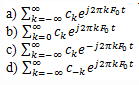Solution:

Explanation: If the given signal is x(t) and F0 is the reciprocal of the time period of the signal and ck is the Fourier coefficient then the Fourier series representation of x(t) is given as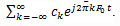QUESTION: 2

### Which of the following is the equation for the Fourier series coefficient?

Solution:

Explanation: When we apply integration to the definition of Fourier series representation, we get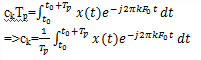QUESTION: 3

### Which of the following is a Dirichlet condition with respect to the signal x(t)?

Solution:

Explanation: For any signal x(t) to be represented as Fourier series, it should satisfy the Dirichlet conditions which are x(t) has a finite number of discontinuities in any period, x(t) has finite number of maxima and minima during any period and x(t) is absolutely integrable in any period.

QUESTION: 4

The equation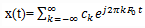is known as analysis equation.

Solution:

Explanation: Since we are synthesizing the Fourier series of the signal x(t), we call it as synthesis equation, where as the equation giving the definition of Fourier series coefficients is known as analysis equation.

QUESTION: 5

Which of the following is the Fourier series representation of the signal x(t)?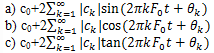d) None of the mentioned

Solution:

Explanation: In general, Fourier coefficients ck are complex valued. Moreover, it is easily shown that if the periodic signal is real, ck and c-k are complex conjugates. As a result
ck=|ck|ejθkand ck=|ck|e-jθk
Consequently, we obtain the Fourier series as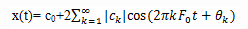QUESTION: 6

The equation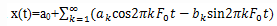is the representation of Fourier series.

Solution:

Explanation: cos(2πkF0 t+θk)= cos2πkF0 t.cosθk-sin2πkF0 t.sinθk
θk is a constant for a given signal.
So, the other form of Fourier series representation of the signal x(t) is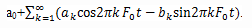QUESTION: 7

What is the spectrum that is obtained when we plot |ck |2 as a function of frequencies kF0, k=0,±1,±2..?

Solution:

Explanation: When we plot a graph of |ck |2 as a function of frequencies kF0, k=0,±1,±2… the following spectrum is obtained which is known as Power density spectrum.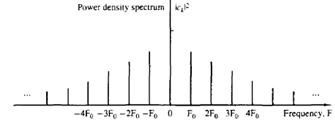QUESTION: 8

What is the spectrum that is obtained when we plot |ck| as a function of frequency?

Solution:

Explanation: We know that, Fourier series coefficients are complex valued, so we can represent ck in the following way.
ck=|ck|ejθk
When we plot |ck| as a function of frequency, the spectrum thus obtained is known as Magnitude voltage spectrum.

QUESTION: 9

What is the equation of the Fourier series coefficient ck of an non-periodic signal?

Solution:

Explanation: We know that, for an periodic signal, the Fourier series coefficient is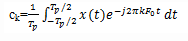If we consider a signal x(t) as non-periodic, it is true that x(t)=0 for |t|>Tp/2. Consequently, the limits on the integral in the above equation can be replaced by -∞ to ∞. Hence,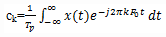QUESTION: 10

Which of the following relation is correct between Fourier transform X(F) and Fourier series coefficient ck

Solution:

Explanation: Let us consider a signal x(t) whose Fourier transform X(F) is given as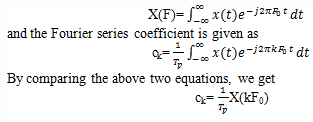Use Code STAYHOME200 and get INR 200 additional OFF Use Coupon Code